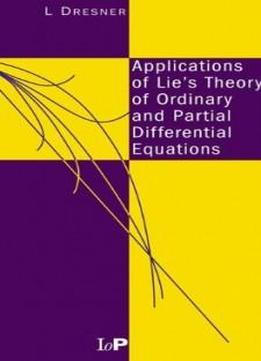# Applications Of Lie's Theory Of Ordinary And Partial Differential Equations by L Dresner / 1998 / English / PDF

Lie's group theory of differential equations unifies the many ad hoc methods known for solving differential equations and provides powerful new ways to find solutions. The theory has applications to both ordinary and partial differential equations and is not restricted to linear equations. Applications of Lie's Theory of Ordinary and Partial Differential Equations provides a concise, simple introduction to the application of Lie's theory to the solution of differential equations. The author emphasizes clarity and immediacy of understanding rather than encyclopedic completeness, rigor, and generality. This enables readers to quickly grasp the essentials and start applying the methods to find solutions. The book includes worked examples and problems from a wide range of scientific and engineering fields.

Lie's group theory of differential equations unifies the many ad hoc methods known for solving differential equations and provides powerful new ways to find solutions. The theory has applications to both ordinary and partial differential equations and is not restricted to linear equations. Applications of Lie's Theory of Ordinary and Partial Differential Equations provides a concise, simple introduction to the application of Lie's theory to the solution of differential equations. The author emphasizes clarity and immediacy of understanding rather than encyclopedic completeness, rigor, and generality. This enables readers to quickly grasp the essentials and start applying the methods to find solutions. The book includes worked examples and problems from a wide range of scientific and engineering fields.

views: 372